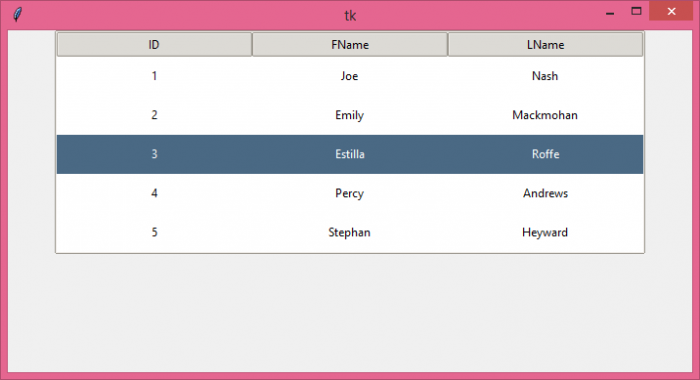# How can I set the row height in Tkinter TreeView?

The treeview widget in Tkinter provides a way to represent the data in a hierarchical structure. With the Treeview widget, we can insert our data in the form of a table. The table can have rows and columns in which we can insert the data instantly.

We can also configure the properties of the treeview widget such as its color, size, column width, height, row width & height, etc. To set the row height of the Treeview widget, you can create an instance of ttk themed widget where you can specify the rowheight property. The rowheight property will add internal padding to each row in the table.

## Example

# Import the required libraries
from tkinter import *
from tkinter import ttk

# Create an instance of tkinter frame
win=Tk()

# Set the size of the tkinter window
win.geometry("700x350")
s=ttk.Style()
s.theme_use('clam')

s.configure('Treeview', rowheight=40)

tree.column("# 1",anchor=CENTER)
tree.column("# 2", anchor=CENTER)
tree.column("# 3", anchor=CENTER)

# Insert the data in Treeview widget
tree.insert('', 'end',text="1",values=('1', 'Joe','Nash'))
tree.insert('', 'end',text="2",values=('2', 'Emily','Mackmohan'))
tree.insert('', 'end',text="3",values=('3', 'Estilla','Roffe'))
tree.insert('', 'end',text="4",values=('4', 'Percy','Andrews'))
tree.insert('', 'end',text="5",values=('5', 'Stephan','Heyward'))

tree.pack()

win.mainloop()

## Output

If we run the above code, it will display a window with a table and some data in it. In the given table, each row has an assigned row height.